﻿ Excel一个单元格字符有几行？ - 永恒君的百宝箱

# Excel一个单元格字符有几行？

80℃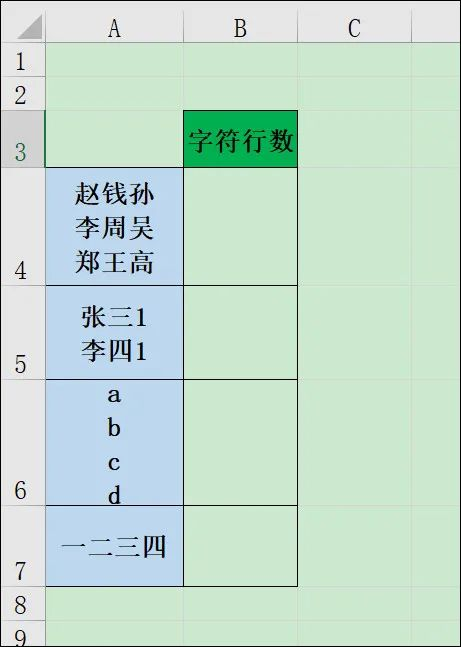``````=LEN(A4)
``````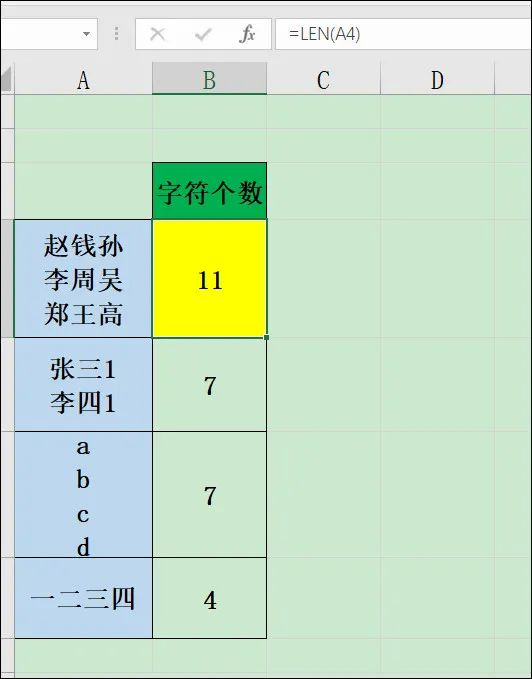``````=LEN(SUBSTITUTE(A4,CHAR(10),""))
``````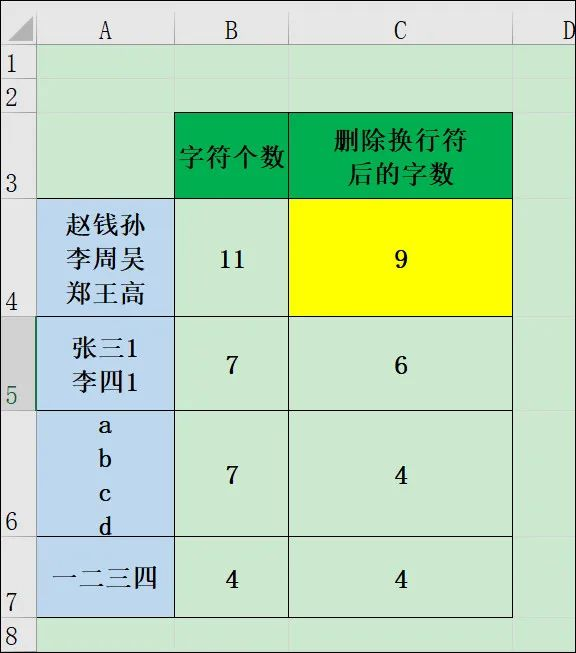``````=B4-C4
``````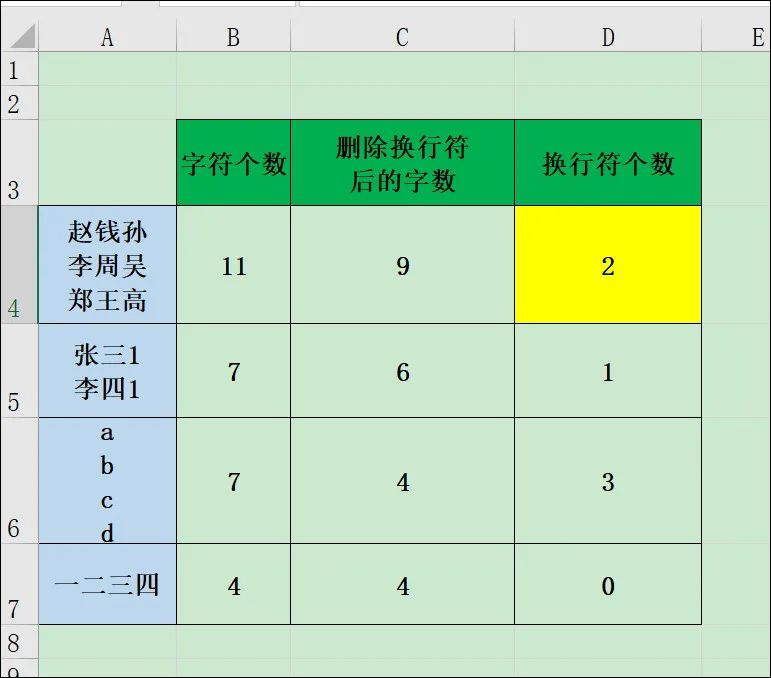``````=D4+IF(LEN(A4)>0,1,0)
``````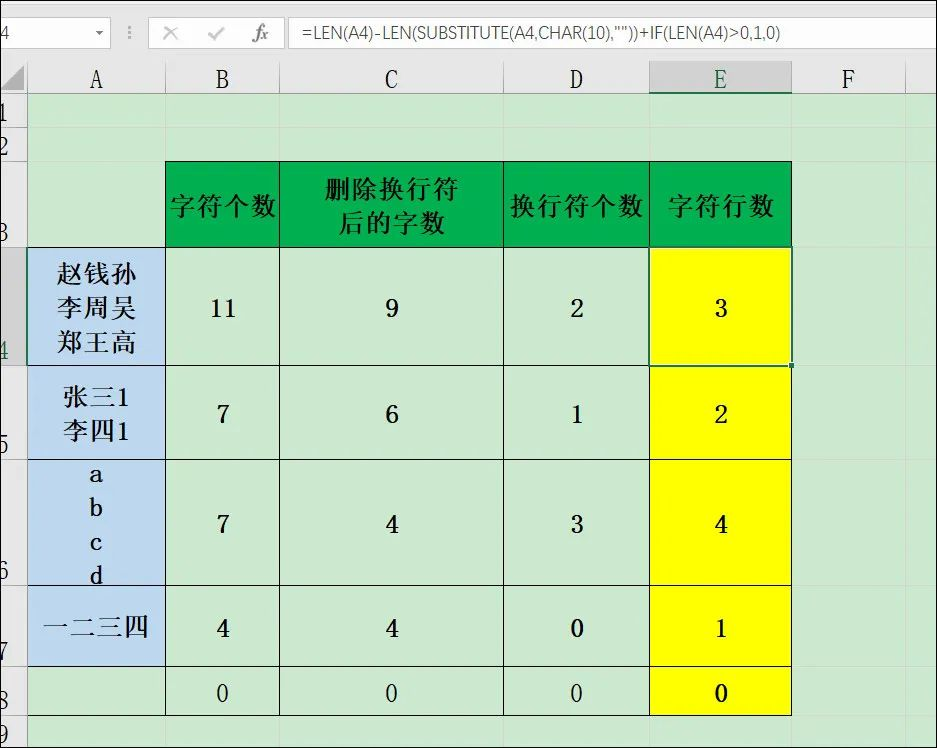``````=LEN(A4)-LEN(SUBSTITUTE(A4,CHAR(10),""))+IF(LEN(A4)>0,1,0)
``````